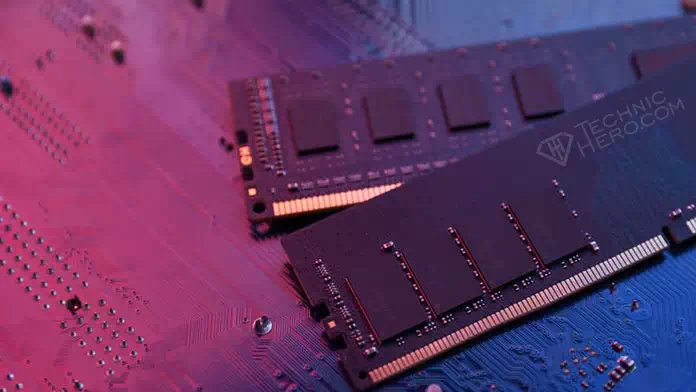What is Memory Rank? What Do Terms Like 1Rx8, 1Rx16, 2Rx4, 2Rx8, 2Rx16, 4Rx4, 4Rx8, 4Rx16 Mean? What is the difference between 1Rx8 and 2Rx8 RAM?

We wonder what terms such as 1Rx8 and 2Rx8, which are terms we encounter while buying ram or upgrading our computer’s memory, mean. Sometimes we are investigating whether rams with different terms will work together and cause problems. Let us now explain what these terms mean, both simply and technically.

## What is Memory Rank?

Memory Rank is a very technical issue. To put it simply, Memory rank i.e. terms like 1Rx8 and 2Rx8 refer to the design of the memory and how many chips are used etc. It is a related topic. In a term like 1Rx8, 1R and x8 represent two separate features. 1R part: Represents the number of memory rows (how many data blocks are in Ram). The x8 part represents how many bit chips are used.

Data blocks will be 64bits long. (In some systems that support ECC, a total of 72bits is obtained by adding 8bits.) As you can see in the picture below, each row is designed to be 64bit. If all chips are 64bit, that’s one row. If there are 2 64bits, it means that there are two ranks (Dual Rank). If there are 4 64bit blocks, it means that it is a Quad Rank ram.

### What is the Difference between 1Rx8 (Single Rank) and 2Rx8 (Dual Rank)?

A Dual Rank memory can be compared to two memory units combined in a single module. For example, if the motherboard supports it, 2 Dual Rank 8GB memory modules can be used instead of 4 Single Rank 4GB memory modules. In this case, you cannot use the other two free slots. Because theoretically, you will have installed 4 ram. In another example: Let’s say your motherboard has 8gb Single Rank support. If you install 2 pieces of 4gb Double Rank Ram, the system will not see the 2nd Ram because it cannot address it. Because your motherboard supports 2 single rank ram, you will be as if you have installed 4 single ram.

Systems that support dual rank do not support single rank, because these systems are not produced for single rank addressing. So if you are going to upgrade next to a ram like 2Rx4, 2Rx8. The Ram you will receive should be 2Rx. Therefore, it will be better if the ram you buy is of the same rank type as the first ram.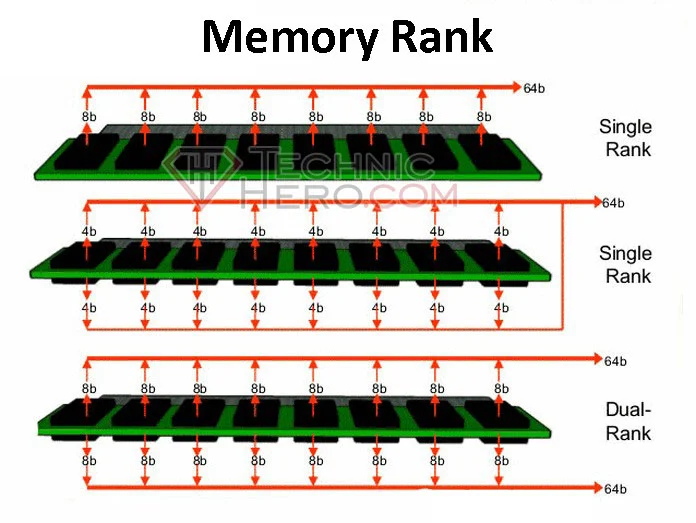The presence of chips on both sides of the memory or the presence of chips on one side is not a criterion for Ram to be Single or Double Rank. Single Rank and Double Rank are all about the design of the ram as fabrication.

### What is Memory Rank in Detail?

Memory Rank, was created by JEDEC company, one of the standard groups of the memory industry. This term was coined to distinguish between the number of memory chips on a component or memory chip and the number of memory banks in a module.

A memory rank is a block or area of data created using some or all of the memory chips. A queue is a 64-bit wide data block. On systems that support Error Correction Code (ECC), an additional 8 bits are added making the data block 72 bits wide. Depending on how a memory module is designed, it can have one, two, or four 64-bit wide data fields (or 72-bit wide in the case of ECC modules). This is called single-row, double-row. Single Row, Double Row and Quad Row. It states on memory labels as 1Rx8 or 2Rx8, 4Rx4 or similar.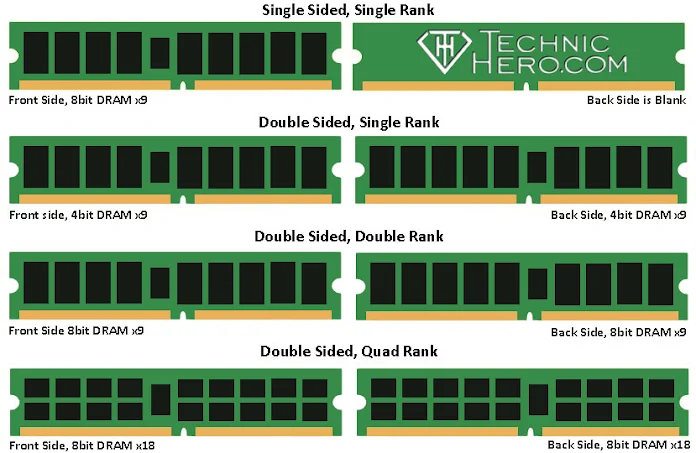#### Single Rank Memory

• (1Rx8) Single Rank, Single Row: In this model with a single data block, one side of the Ram may be empty. In total; Desktop memories have 9, Laptop Memory has 8 8bit chips. This is 9×8=72(64+8)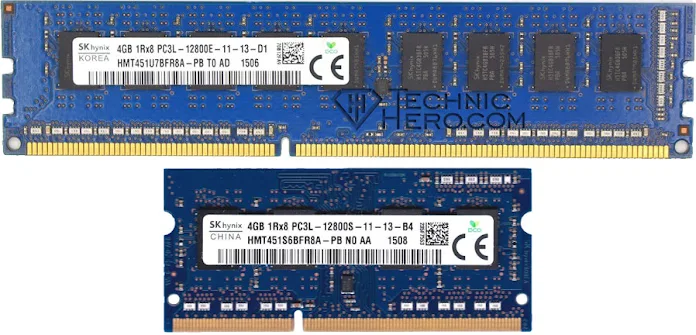• (1Rx4) Double-Sided, Single Row: In this type of Ram, there are 9 pieces of RAM on both sides, a total of 18 pieces of 4bit chips. This equals 18×4=72bits• (1Rx16) Single Sided, Single Row: In this model, which is called Single Rank, that is, it has a single data block, one side of the Ram may be empty. On the other side, there are 4 16-bit chips. This is 4×16=64bit.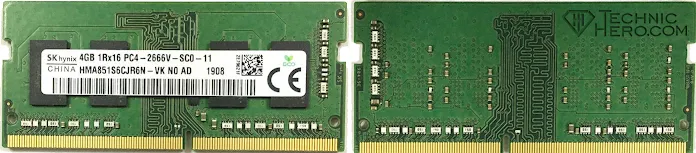#### Dual Rank Memory

• (2Rx8) Double Sided, Double Row: This type of Ram has Dual Rank, two data blocks. There are 18 chips and 2 rows in total, 9x8bit=72 bits on the front of the RAM, and 9x8bits=72 bits on the other side. On laptops it is 8×8=64.• (2Rx4) Double Sided, Double Row: This type of Ram has Dual Rank, two data blocks. There are 2 rows of 18x4bits=72 bits on the front of the RAM, and 18x8bits=72 bits on the other side.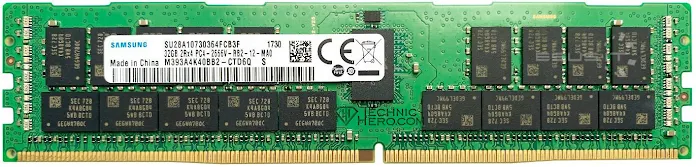• (2Rx16) Double Sided, Double Row: This type of Ram has Dual Rank, two data blocks. There are 2 rows of 4x16bit=64 bits on the front of the RAM, and 4x16bits=64 bits on the other side. That’s 128 bits in total. Or There are also models with 8 chips on a single face.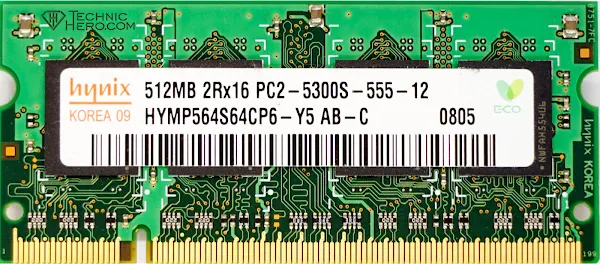• (4Rx8) Double Sided, Four Rows: These memories have Quad Rank, that is, 4 data blocks. Both sides have a total of 36 chips. In other words, there are 4 (8×8=64)+8=72 bits. 4×72=288 bits in total.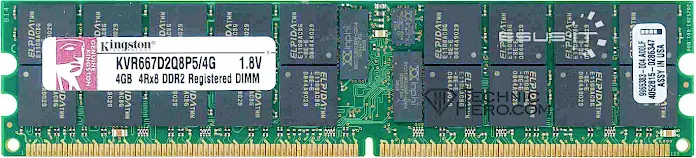• (4Rx4) Double-Sided, Four Rows: Theoretically 64ad x 4bit = 256bit in these memories. However, as a design, you cannot see 64 chips on the ram. Instead, it contains 16 chips each equivalent to 4 16-bit chips. So 16 x (4×4) = 256bit chip. Or chips with different values that take up less space can also be produced. For example: It can be produced in the form of 36 x (2×4)=288bit rams. In this, 36 chips of 2 bits each are used.#### How to Learn Memory Rank?

The Rank type of the memories is usually written on the label on them. However, some memories may not be specified on the label. Or you may want to learn the Rank type of a ram installed in the computer. For this: You can use the CPU-Z program. Switch to the “SPD” tab in the program, where it says “Ranks”, you can learn the Ranking type of your memory.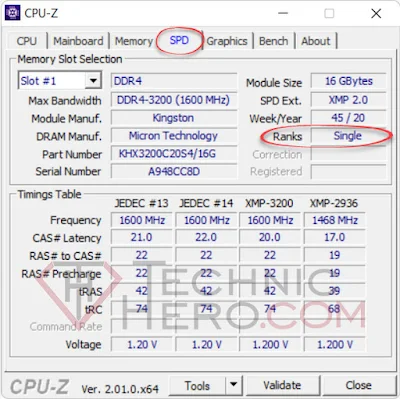Subscribe
Notify of0 Comment
Inline Feedbacks